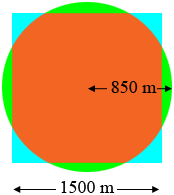SEARCH HOMEMath Central Quandaries & QueriesQuestion from rajpal: i m trying to calculate an area required to hold 4.4 mln cubic metres of volume. I used square and I get below result A box shape that has dimensions 1500 meters (1.5km) by 1500 meters (1.5km) by 2 meters depth has a volume of: = 4,500,000 cubic meters (4.5 mln cubic meters) but when I use a cylinder shape, i get below result A cylindrical shape that has a radius of 850 meters and a depth of 2 meters has a volume of: = 4,539,600 cubic meters (4.54 mln cubic meters approx) why is it that the cylinder, though of same depth holds more volume than a square? kindly clarify.Hi,

I drew a diagram, roughly to scale, of a square of side length 1500 m and overlaid it by a circle of radius 850 m.Part of the square is outside the circle, shaded blue, and part of the circle is outside the square, shaded green.

The area of the square is

$1500 \times 1500 = 2,250,000 \mbox{ square metres}$

and the circle is

$\pi \times 850^2 = 2,269,800.69 \mbox{ square metres.}$

Since the area of the circle is larger than the area of the square the sum of the areas of the green parts of the diagram is larger than the sum of the areas of the parts of the diagram shaded blue.

I hope this helps,
PennyMath Central is supported by the University of Regina and the Imperial Oil Foundation.